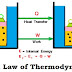State first law of thermodynamics and prove ΔE = qv.

First Law of Thermodynamics

"This law is also called the law of conservation of energy. This law is stated as, "energy of the universe is constant".

(OR)

"Energy can neither be created nor destroyed but can change from one form to another."

In other words, a system cannot destroy or create energy. However, it can exchange energy with its surrounding in the form of heat or work. Thus the energy change is the sum of both heat and work so that the total energy of the system and its surroundings remains constant.

Consider a gas enclosed in a cylinder having a piston. Suppose the internal energy of the system is E1. A quantity of heat q is given to the system and work W is done on the piston to keep it in its original position. During these operations, the internal energy of the system changes to E2, the change in internal ΔE is given by the following equation, which is the mathematical form of the first law of thermodynamics.

E2 - E =  ΔE = q + W

ΔE  = q + W

Sign of q will be positive when heat is supplied to the system and q is negative when heat flows out side across the boundary. W is negative when work is done by the system and W is positive when work is done in the system. Pressure volume work is given mathematically as:

Work = Force  x  Distance

An external pressure P exerted by a force F, spreads over the area A, as pressure is force per unit area.

P  =  F/A    or     F  =  P x A

The volume of the gas in the cylinder is equal to cross-section area A multiplied by the height of the column of the gas h.

V  =  A  x  h

Now, let us assume that the gas expands and does work by pushing the piston against external pressure, "A" remains the same but "h" changes.

ΔV  =  V2  -  V1

ΔV  =  Ahf  -  Ahi

ΔV  =  A (hf - hi)

ΔV  =  A  Δh

Work done by expansion of gas against constant pressure is given by

W  =  -F  x  Δh

W  =  -P  x  A  x  Δh

W  =  -P ΔV

The negative sign indicates that work is done by the system on the surrounding. So first law of thermodynamics can be written as:

ΔE  =  q  -  P ΔV

Energy changes at constant volume

If the volume of gas does not change, no work is done, (ΔV  =  0). By applying the first law of thermodynamics.

ΔE  =  qv  -  P ΔV

ΔE  =  qv  -  0      (ΔV = 0)

ΔE  =  qv

So the increase of heat at constant volume (qv) increases only the internal energy (ΔE) of the system and work done is zero.

First Law of Thermodynamics (ΔE = qv)Reviewed by SaQLaiN HaShMi on 6:03 AM Rating: 5